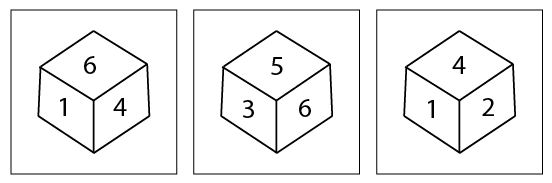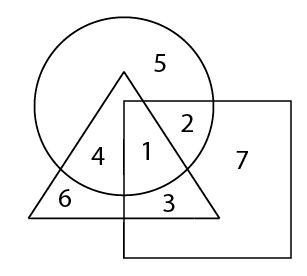# SSC CGL 2013 Tier 1 21 April Shift 2

Instructions

For the following questions answer them individually

Question 181

Which of the following interchange of signs would make the given equation correct ?
2 × 3 + 6 - 12 ÷ 4= 17

Question 182

‘B’ stands for addition, ‘Cr’ stands for subtraction, ‘E’ stands for multiplication, ‘C’ stands for division, ‘D’ stands for equal to, ‘A’ stands for greater than, stands for less than. In each of the four alternatives, only one expression is correct according to the letter symbol. Identify that expression.

Question 183

Identify the symbols to be inserted to make the expression correct.
24 Δ 4 Δ 5 Δ 4

Question 184

6 boys A, B, C, D, E, F are sitting in a row facing West. D is between A and C. B is just right of C but left of F. E is not at the right end. Who is at the right end ?

Question 185

Y is in the East of × which is in the North of Z. If P is in the South of Z, then in which direction of Y is P ?

Question 186

Kate walks 4 km towards South. She then turns towards her left and walks 8 km more. After that she turns left again and walks another 8 km. Here, she meets her friend coming from the opposite direction and they both stop here. Which direction would she be facing ?

Instructions

In the following questions, two statements are given followed by two/four conclusions I, II, III and IV. You have to consider the two statements to be true even if they seem to be at variance from commonly known facts. You have to decide which of the given conclusions, if any, follow from the given statements.

Question 187

Statements :
I. Some birds are clouds.
II. Horse is a bird.
Conclusions :
I. Some clouds are birds.
II. Horse is not a cloud.

Question 188

Statements :
I. Ravi has five pens.
II. No one else in the class has five pens.
Conclusions :
I. All students in the class have pens.
II. All students in the class have five pens each.
III. Some of the students have more than five pens.
IV. Only one student in the class has exactly five pens.

Instructions

For the following questions answer them individually

Question 189

Three positions of a dice are given. Find out which number is found opposite the number 2 in the given cube.Question 190

In the following figure Circle represents hardworking. Triangle represents sincere and Square represents intelligent. Find out the hardworking who are intelligent but not sincere.OR